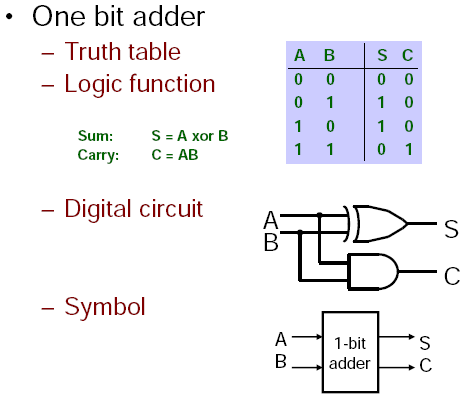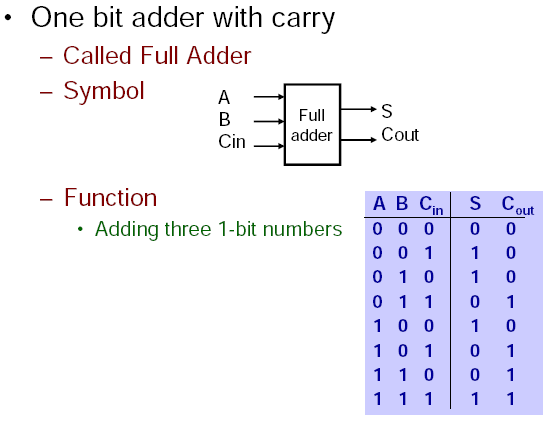#tianjara.net |Andrew Harvey's Blog

14th April 2009

Just some notes on using ADD and ADC (and also a not on compare and branch instructions at the end). (Thanks also to my lab partner who helped me with some of this stuff.)

The registers for AVR are 8 bits long, as noted if I want to store a 16 bit number I need to use two registers, one will store the low byte and the other will store the high byte. eg the 16 bit binary number a = 1111111100000000 (as big endian) would be stored as a_high = 11111111, a_low = 00000000.

Another thing that I've learnt is how addition of multi byte numbers in AVR works. AVR has the instructions ADD (add without carry) and ADC (add with carry). Lets look at a simple case first.

ldi r16, 0b10101010 //the 0b indicates binary (\$ or 0x for hex, nothing for decimal, 0 for octal). loads the binary number 10101010 into register 16.
ldi r17, 0b10101010
add r16, r17

What happens here is,

  10101010
+ 10101010
01010100
c c c c

The carry bit is set, and hence and overflow has occurred. The actual answer is 101010100 but this has 9 bits and cannot fix in the 8 bits of space we have for the result to be stored into r16. Here we used the ADD instruction this means (at least to the best of my understanding, please correct me if I'm wrong) that we ignore what is originally in the carry flag (part of the status register). If we used ADC and the carry flag was set to 1 then when we add the right most bit as above 0+0 = 0 but we have a carry so we would get a 1 in that last bit. We can use this to do multi byte arithmetic.

Say we have,

//a is a 16 bit number 1010101010101010
ldi al, 0b10101010
ldi ah, 0b10101010
//b is a 16 bit number 1010101010101010
ldi bl, 0b10101010
ldi bh, 0b10101010

//to do a+b (and store the result in a) we do
adc ah, bh

We can then extend this to as many bytes as we want. Say we have a 3 byte number spread over the registers of a_0, a_1, a_2 and another 3 byte number in the registers b_0, b_1, b_2. We could add them like this,

add a_0, b_0
adc a_2, b_2

The result would be spread over a_0, a_1 and a_2 (remember though that we can still overflow this).

It was also enlightening (though also a nice reminder) to see how this low level addition works. (when doing 1bit addition (without carry), a + b, the sum = a xor b and the carry = a and b.) (see picture below)(Credit: Annie Guo, Basics of Digital Systems, COMP2121 Notes)

This concept can similarly be applied to other instructions. For example,

cp a, b
breq label

will compare a and be and branch to the label label if a is equal to b (and if not continue on executing the subsequent instructions). However what if you wanted to compare if a two byte number was equal to a two byte number? Sure you could just repeat the code one time for the low byte and another for the high byte but you can also do this,

cp al, bl
cpc ah, bh
breq label

These cp and cpc instructions use some trickery (well not really but I didn't initially see how this worked, though its quite obvious now) where they modify some flags based on the result of the comparison and then the branch instructions (breq, brne, brlo...) look at the respective flag to see if they should branch or not.

Tags: avr, comp2121, computing.